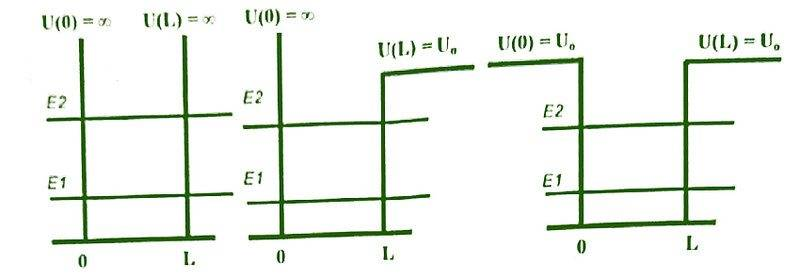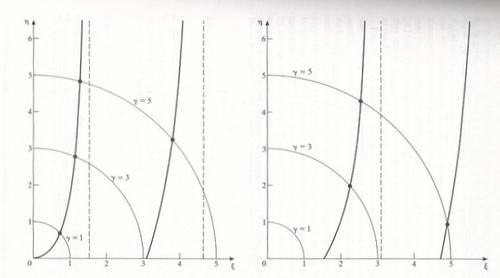# What is the number of confined states in these potential wells?

patric44
Homework Statement:
what is is maximum and minimum number of confined states within these potential wells ?
Relevant Equations:
UL>(pi^2)(hbar^2)/8me , the condition for existence of states in the semi infinite well
hi guys
i came across this question about the maximum and minimum number of bound states that can be confined in these potential wells
1- infinite potential well
2- semi infinite potential well (from one side)
3 - finite potential well
i think i have a good idea about the minimum number of states i guess :
1- the infinite has minimum of 1 state it could always have at least one state
2 - the semi infinite if it were very shallow it could have no states so the min is 0
3 - the finite also always have at least 1 states inside no matter how shallow
is that correct.

and I am not sure what is the maximum in each and shouldn't that be dependent on the exact value of V ,
is there is a formula for that that depend on potential ?

Staff Emeritus
(1) You're surely not writing down the exact problem. "Infinite potential well" could mean many things. Most likely it means 1-dimensional infinite square well, but you shouldn't make us have to guess.

(2) You're not working the problems. You're guessing, and you're asking us if your guess is right.

•vanhees71 and patric44
Keith_McClary
Most likely it means 1-dimensional infinite square well, but you shouldn't make us have to guess.
The answer depends on the dimension.

•patric44
patric44
(1) You're surely not writing down the exact problem. "Infinite potential well" could mean many things. Most likely it means 1-dimensional infinite square well, but you shouldn't make us have to guess.
(2) You're not working the problems. You're guessing, and you're asking us if your guess is right.
I am sorry I should had puten more effort in presenting the problem and my attempt, so here we go :
in the following 1D potential wells as described by the figures :the question was asking to find the maximum and the minimum number of bound states confined within these systems ?
the minimum number can be obtained by looking at the transcendental equation plot for each system, i am taking about the the last two systems , since the infinite well is infinite i guess there is no max and the min is 1 correct me if I am wrong, now for the two systems:the left one is for the finite well and it shows an intersection point (represents a solution) no mater how shallow the well is . so the minimum is 1.
the right one shows no intersection up till a condition of $$UL>\frac{\pi^{2}\hbar^{2}}{8m}$$
so the min is 0.
not sure how to get the maximum number of states , is it by counting the total intersection points
or there is another approach ?